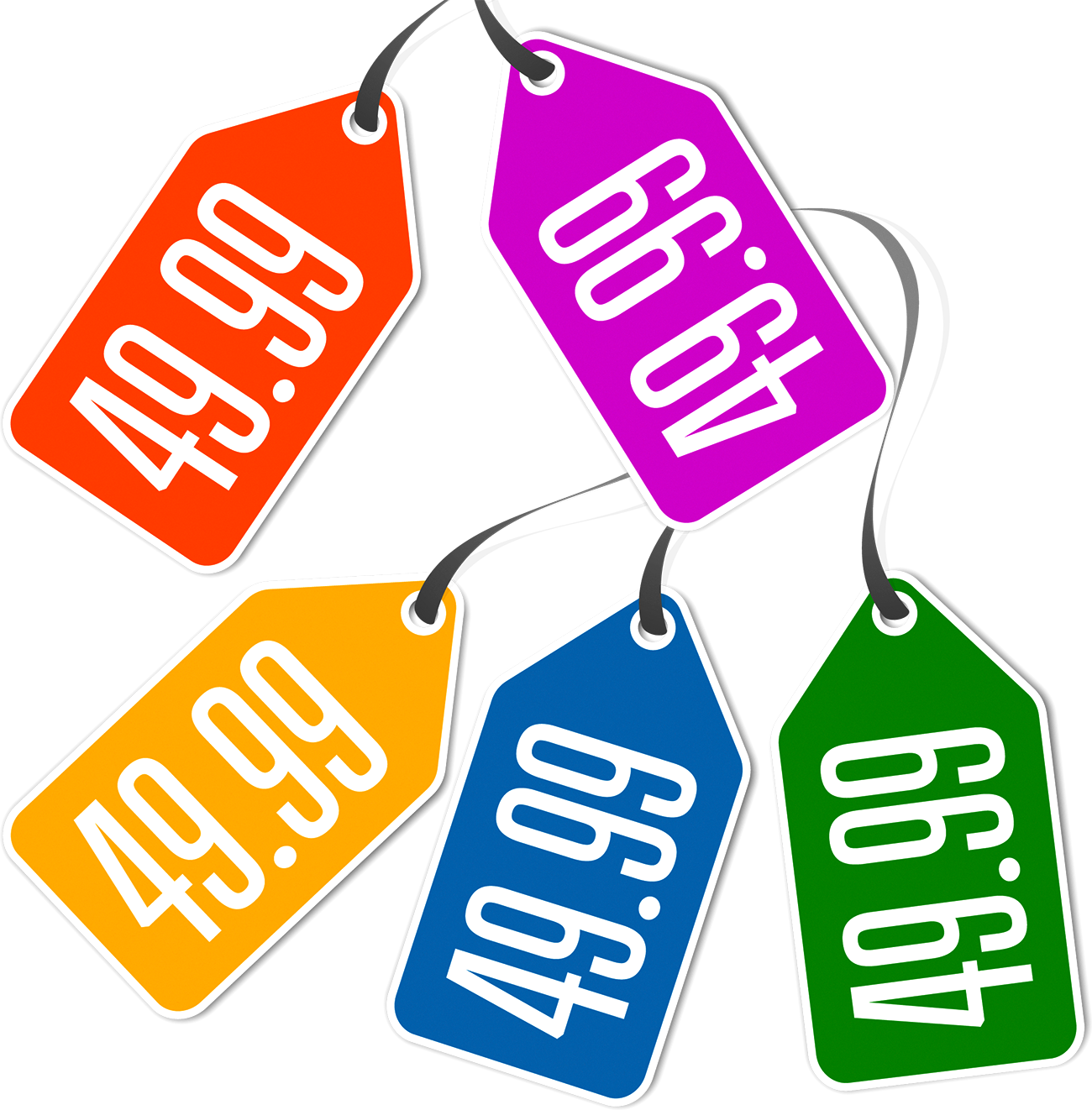• Call Now

1800-102-2727•

# Decimals in Daily Life

Decimals are used in our day to day life. Be it money, length, weight etc., the usage of decimal digits in one’s life is extraordinary. The main goal of the usage of decimal numbers is to acquire more precision. Some real-life instances are when we try to calculate our weight using the weighing machine, and we don’t find the weight in just whole numbers. We will also get decimal digits as a result. In order to completely understand the nature of our weight, we need to know exactly what those decimal numbers mean. This article will discuss the decimal concepts in the three most important fields of our everyday life.## Usage of decimals in everyday life

### Money:

It is almost always impossible not to use decimals while dealing with cash. When we have to convert paisa into rupee or talk about the big amount of cash easily, like 1.5 thousand rupees, 7.5 lakhs, 10.2 lakhs, etc., we all need to use decimals. Imagine if we go to a nearby grocery shop to buy 500 grams of cashew nut, where 1 kg costs 1,205 rupees. So, we have to calculate it for half a kilogram. The total amount of money for half a kilogram would be 602.50 rupees, in which the 602 indicates rupees whereas the .50 indicates paisa. The following is the math involved in this calculation;

1 Rupee = 100 Paise
0.5 Rupee = 50 Paise
602.5 Rupees = 602 Rupees and 50 Paisa

### Weight:

Decimals are a must when we calculate the weight. It is inevitable. For instance, when we go shopping to buy a watermelon, and it is anywhere between 1 kilogram to 3 kilograms, (It cannot always be the whole number), let's assume it is 1.75 kg or 2.5 kg. In such types of scenarios, the shop owner is urged to calculate how much it costs based on its weight,

The below mentioned is the conversion of kilogram into other units,

1 kg = 1000 gram
1 gram = 1000 milligram

Now suppose the watermelon that we want to buy weighs 1.75 kilograms, then he will charge as per the price of 1 kg + (7500/1000) kg of the watermelon.

### Length:

It is necessary to measure the length of an item, and it cannot be only of whole digits but also contains decimals. For example, to measure the length of any given bed with a measuring tape, the length might not necessarily be a whole number. Instead, it may lie somewhere between any two graduations on the tape. In this situation, decimal numbers can be used significantly, and one should know what it means while measuring.

The following is the common unit of conversion in terms of length,

1 km = 1000 m
1 m = 100 cm
1 cm = 10 mm

Now, imagine the length of the bed is 3 m and 50 cm, then it should be represented as (3 + 50/100) m. Therefore, this can also be shortly termed as 3.5 metres.Talk to our expert
Resend OTP Timer =
By submitting up, I agree to receive all the Whatsapp communication on my registered number and Aakash terms and conditions and privacy policy